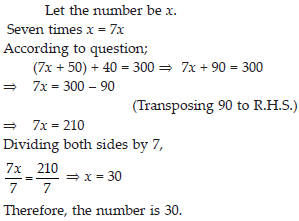# Simple Equations (Maths) Class 7 - NCERT Questions

Q 1.

Complete the last column of the table.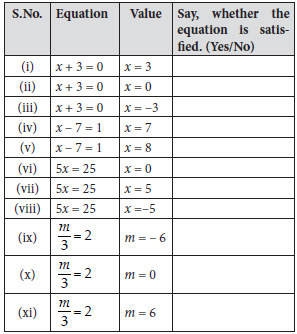SOLUTION:

(i) x + 3 = 0
By putting x = 3
L.H.S. = 3 + 3 = 6 ≠ R.H.S.
Hence, the equation is not satisfied.
(ii) x + 3 = 0
By putting x = 0
L.H.S. = 0 + 3 = 3 ≠ R.H.S.
Hence, the equation is not statisfied.
(iii) x + 3 = 0
By putting x = – 3
L.H.S. = – 3 + 3 = 0 = R.H.S.
Hence, the equation is satisfied.
(iv) x – 7 = 1
By putting x = 7
L.H.S. = 7 – 7 = 0 ≠ R.H.S.
Hence, the equation is not satisfied.
(v) x – 7 = 1
By putting x = 8
L.H.S. = 8 – 7 = 1 = R.H.S.
Hence, the equation is satisfied.
(vi) 5x = 25
By putting x = 0
L.H.S. = 5 × 0 = 0 ≠ R.H.S.
Hence, the equation is not satisfied. (vii) 5x = 25
By putting x = 5
L.H.S. = 5 × 5 = 25 = R.H.S.
Hence, the equation is satisfied.
(viii) 5x = 25
By putting x = – 5
L.H.S. = 5 × (–5) = –25 ≠ R.H.S.
Hence, the equation is not satisfied. (ix) m/3=2
By putting m = – 6
L.H.S.R.H.S.=−=−≠632
Hence, the equation is not satisfied.
(x) m/3=2
By putting m = 0
L.H.S. = 03 = 0 ≠ R.H.S.
Hence, the equation is not satisfied.
(xi) m/3= 2
By putting m = 6
L.H.S. = 63 = 2 = R.H.S
Hence, the equation is satisfied.

Q 2.

Check whether the value given in the brackets is a solution to the given equation or not:
(A) n + 5 = 19 (n = 1)
(B) 7n + 5 = 19 (n = –2)
(C) 7n + 5 = 19 (n = 2)
(D) 4p – 3 = 13 (p = 1)
(E) 4p – 3 = 13 (p = – 4)
(F) 4p – 3 = 13 (p = 0)

SOLUTION:

(A) n + 5 = 19 (n = 1)
Putting n = 1
L.H.S. = n + 5 = 1 + 5 = 6 ≠ 19 = R.H.S.
As L.H.S. ≠ R.H.S.
Therefore, n = 1 is not a solution of the given equation, n + 5 = 19.
(B) 7n + 5 = 19 (n = –2)
Putting n = –2
L.H.S. = 7n + 5 = 7 × (–2) + 5 = –14 + 5
= –9 ≠ 19 = R.H.S.
As L.H.S. ≠ R.H.S.
Therefore, n = –2 is not a solution of the given equation, 7n + 5 = 19.
(C) 7n + 5 = 19 (n = 2)
Putting n = 2
L.H.S. = 7n + 5 = 7 × (2) + 5 = 14 + 5 = 19 = R.H.S.
As L.H.S. = R.H.S.,
Therefore, n = 2 is a solution of the given equation, 7n + 5 = 19.
(D) 4p – 3 = 13 (p = 1)
Putting p = 1
L.H.S. = 4p – 3 = (4 × 1) – 3 = 1 ≠ 13 = R.H.S.
As L.H.S. ≠ R.H.S.,
Therefore, p = 1 is not a solution of the given equation, 4p – 3 = 13.
(E) 4p – 3 = 13 (p = – 4)
Putting p = – 4
L.H.S. = 4p – 3 = 4 × (–4) – 3 = –16 – 3
= –19 ≠ 13 = R.H.S.
As L.H.S. ≠ R.H.S.,
Therefore, p = – 4 is not a solution of the given equation, 4p – 3 = 13.
(F) 4p – 3 = 13 (p = 0)
Putting p = 0
L.H.S. = 4p – 3 = (4 × 0) – 3 = – 3 ≠ 13 = R.H.S. As L.H.S. ≠ R.H.S.,
Therefore, p = 0 is not a solution of the given equation, 4p – 3 = 13.

Q 3.

Solve the following equations by trial and error method:
(i) 5p + 2 = 17
(ii) 3m – 14 = 4

SOLUTION:

(i) 5p + 2 = 17 Putting p = 1
L.H.S. = (5 × 1) + 2 = 7 ≠ R.H.S.
Putting p = 2
L.H.S. = (5 × 2) + 2 = 10 + 2 = 12 ≠ R.H.S.
Putting p = 3
L.H.S. = (5 × 3) + 2 = 17 = R.H.S.
Hence, p = 3 is a solution of the given equation.
(ii) 3m – 14 = 4
Putting m = 4,
L.H.S. = (3 × 4) – 14 = –2 ≠ R.H.S.
Putting m = 5,
L.H.S. = (3 × 5) – 14 = 1 ≠ R.H.S.
Putting m = 6,
L.H.S. = (3 × 6) – 14 = 18 – 14 = 4 = R.H.S.
Hence, m = 6 is a solution of the given equation.

Q 4.

Write equations for the following statements:
(i) The sum of numbers x and 4 is 9.
(ii) 2 subtracted from y is 8.
(iii) Ten times a is 70.
(iv) The number b divided by 5 gives 6.
(v) Three-fourth of t is 15.
(vi) Seven times m plus 7 gives 77.
(vii) One-fourth of a number x minus 4 gives 4.
(viii) If you take away 6 from 6 times y, you get 60.
(ix) If you add 3 to one-third of z, you get 30.

SOLUTION: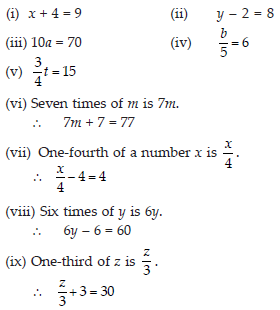Q 5.

Write the following equations in statement form: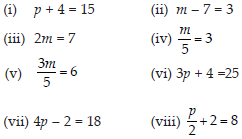SOLUTION:

(i) The sum of p and 4 is 15.
(ii) 7 subtracted from m is 3.
(iii) Twice of a number m is 7.
(iv) One-fifth of m is 3.
(v) Three-fifth of m is 6.
(vi) Three times of a number p, when added to 4, gives 25.
(vii) When 2 is subtracted from four times of a number p, it gives 18.
(viii) When 2 is added to half of a number p, it gives 8.

Q 6.

Set up an equation in the following cases:
(i) Irfan says that he has 7 marbles more than five times the marbles Parmit has. Irfan has 37 marbles. (Take m to be the number of Parmit's marbles.)
(ii) Laxmi's father is 49 years old. He is 4 years older than three times Laxmi's age. (Take Laxmi’s age to be y years.)
(iii) The teacher tells the class that the highest marks obtained by a student in her class is twice the lowest marks plus 7. The highest score is 87. (Take the lowest score to be l.)
(iv) In an isosceles triangle, the vertex angle is twice either base angle. (Let the base angle be b in degrees. Remember that the sum of angles of a triangle is 180°.)

SOLUTION:

(i) Let Parmit has m marbles.
Number of marbles Irfan has = 5 × Number of marbles Parmit has + 7 ∴ 5 × m + 7 = 37 ⇒ 5m + 7 = 37
(ii) Let Laxmi be y years old.
Laxmi’s father’s age = 3 × Laxmi’s age + 4 ∴ 49 = 3 × y + 4 ⇒ 3y + 4 = 49
(iii) Let the lowest marks be l.
Highest marks = 2 × Lowest marks + 7 ∴ 87 = 2 × l + 7 ⇒ 2l + 7 = 87
(iv) An isosceles triangle has two of its angles of equal measure.
Let base angle be b.
Vertex angle = 2 × Base angle = 2b
Sum of all interior angles of a triangle = 180°
∴ b + b + 2b = 180° ⇒ 4b = 180°

Q 7.

Give first the step you will use to separate the variable and then solve the equation:
(A) x – 1 = 0
(B) x + 1 = 0
(C) x – 1 = 5
(D) x + 6 = 2
(E) y – 4 = – 7
(F) y – 4 = 4
(G) y + 4 = 4
(H) y + 4 = – 4

SOLUTION:

(A) x – 1 = 0 On adding 1 to both sides of the given equation, we obtain
x – 1 + 1 = 0 + 1 ⇒ x = 1
(B) x + 1 = 0
On subtracting 1 from both sides of the given equation, we obtain
x + 1 – 1 = 0 – 1 ⇒ x = – 1
(C) x – 1 = 5
On adding 1 to both sides of the given equation, we obtain
x – 1 + 1 = 5 + 1 ⇒ x = 6
(D) x + 6 = 2
On subtracting 6 from both sides of the given equation, we obtain
x + 6 – 6 = 2 – 6 ⇒ x = – 4
(E) y – 4 = – 7
On adding 4 to both sides of the given equation, we obtain
y – 4 + 4 = – 7 + 4 ⇒ y = – 3
(F) y – 4 = 4
On adding 4 to both sides of the given equation, we obtain
y – 4 + 4 = 4 + 4 ⇒ y = 8
(g) y + 4 = 4
On subtracting 4 from both sides of the given equation, we obtain
y + 4 – 4 = 4 – 4 ⇒ y = 0
(h) y + 4 = – 4
On subtracting 4 from both sides of the given equation, we obtain
y + 4 – 4 = – 4 – 4 ⇒ y = – 8

Q 8.

Give first the step you will use to separate the variable and then solve the equation: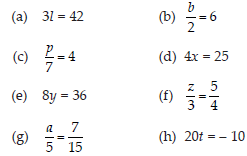SOLUTION:Q 9.

Give the steps you will use to separate the variable and then solve the equation: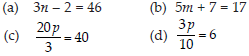SOLUTION: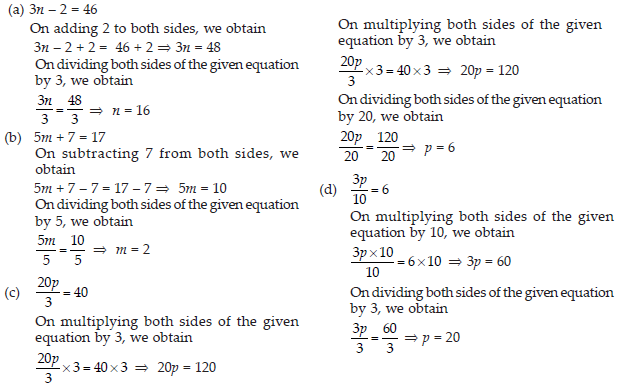Q 10.

Solve the following equations: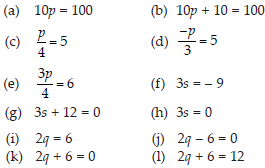SOLUTION: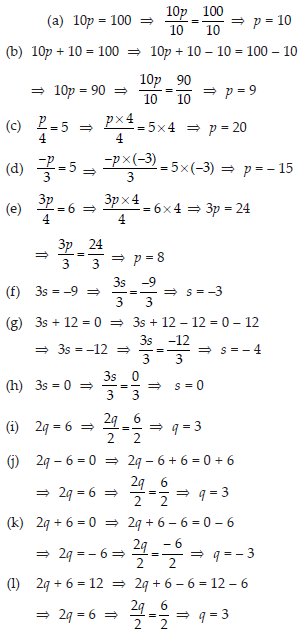Q 11.

Solve the following equations.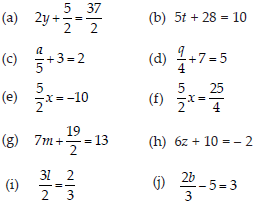SOLUTION: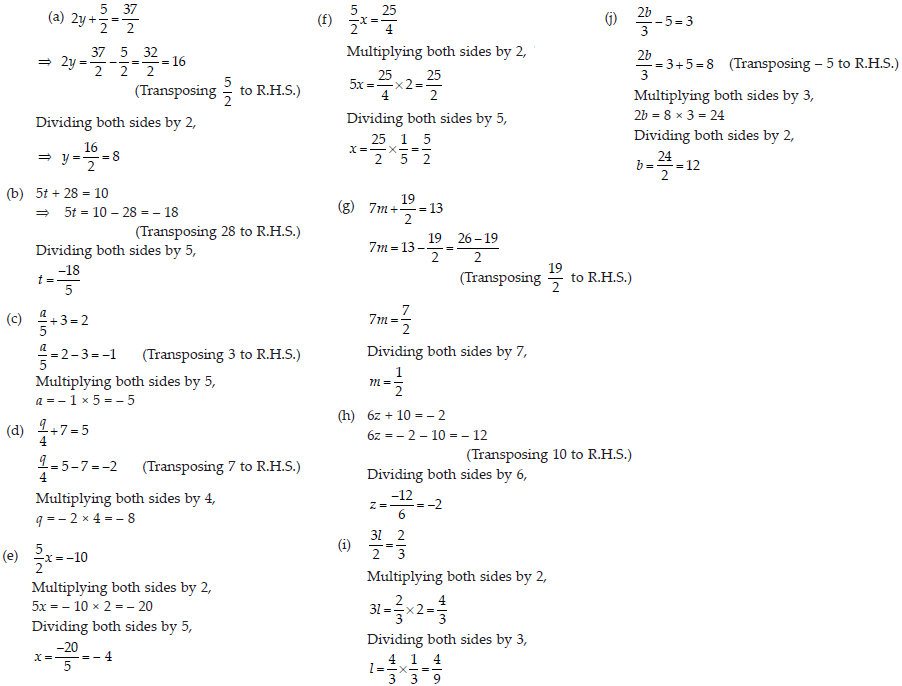Q 12.

Solve the following equations.
(A) 2(x + 4) = 12
(B) 3(n – 5) = 21
(C) 3(n – 5) = – 21
(D) – 4(2 + x) = 8
(E) 4(2 – x) = 8

SOLUTION: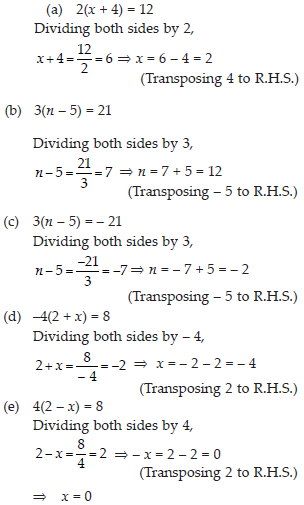Q 13.

Solve the following equations :
(A) 4 = 5(p – 2)
(B) – 4 = 5(p – 2)
(C) 16 = 4 + 3(t + 2)
(D) 4 + 5(p – 1) = 34
(E) 0 = 16 + 4(m – 6)

SOLUTION: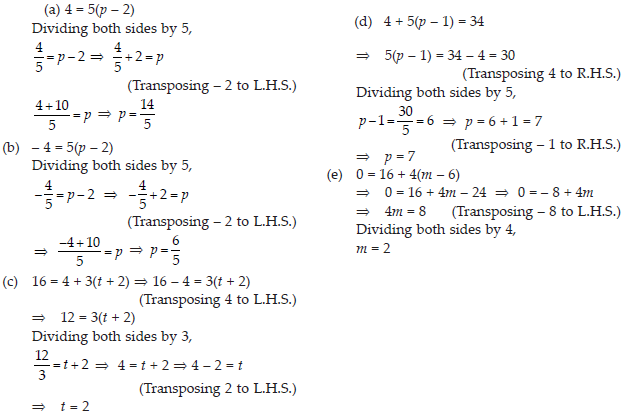Q 14.

(A) Construct 3 equations starting with x = 2.
(B) Construct 3 equations starting with x = – 2.

SOLUTION: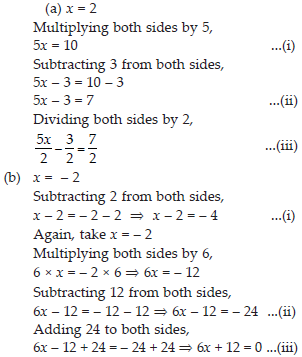Q 15.

Set up equations and solve them to find the unknown numbers in the following cases:
(A) Add 4 to eight times a number; you get 60.
(B) One-fifth of a number minus 4 gives 3.
(C) If I take three-fourths of a number and add 3 to it, I get 21.
(D) When I subtracted 11 from twice a number, the result was 15.
(E) Munna subtracts thrice the number of notebooks he has from 50, he finds the result to be 8.
(F) Ibenhal thinks of a number. If she adds 19 to it and divides the sum by 5, she will get 8.
(G) Anwar thinks of a number. If he takes away 7 from 52 of the number, the result is 23.

SOLUTION: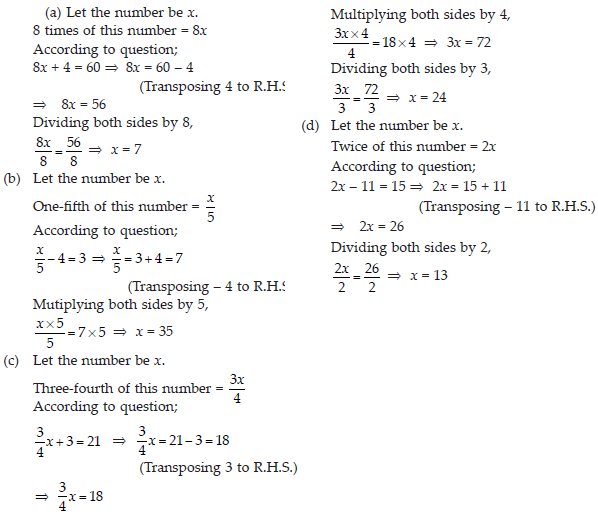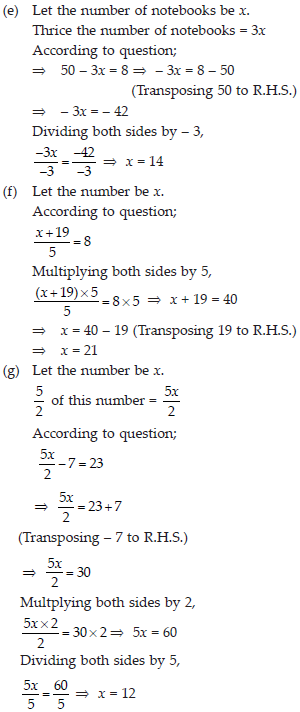Q 16.

Solve the following:
(A) The teacher tells the class that the highest marks obtained by a student in her class is twice the lowest marks plus 7. The highest score is 87. What is the lowest score?
(B) In an isosceles triangle, the base angles are equal. The vertex angle is 40°. What are the base angles of the triangle? (Remember, the sum of three angles of a triangle is 180°).
(C) Sachin scored twice as many runs as Rahul. Together, their runs fell two short of a double century. How many runs did each one score?

SOLUTION: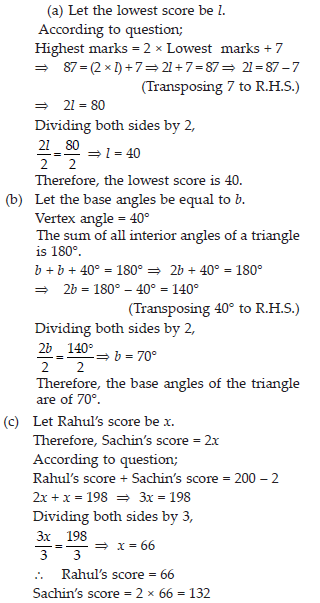Q 17.

Solve the following:
(i) Irfan says that he has 7 marbles more than five times the marbles Parmit has. Irfan has 37 marbles. How many marbles does Parmit have?
(ii) Laxmi’s father is 49 years old. He is 4 years older than three times Laxmi’s age. What is Laxmi’s age?
(iii) People of Sundargram planted trees in the village garden. Some of the trees were fruit trees. The number of non-fruit trees were two more than three times the number of fruit trees. What was the number of fruit trees planted if the number of non-fruit trees planted was 77?

SOLUTION: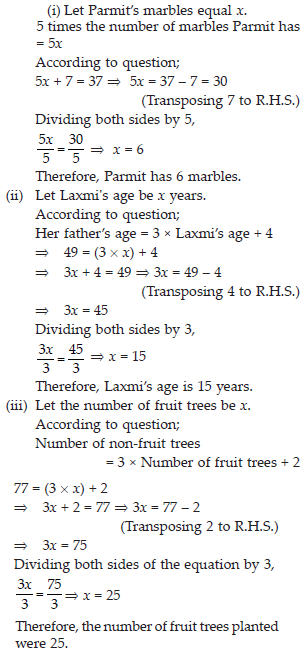Q 18.

Solve the following riddle:
I am a number,
Tell my identity!
Take me seven times over